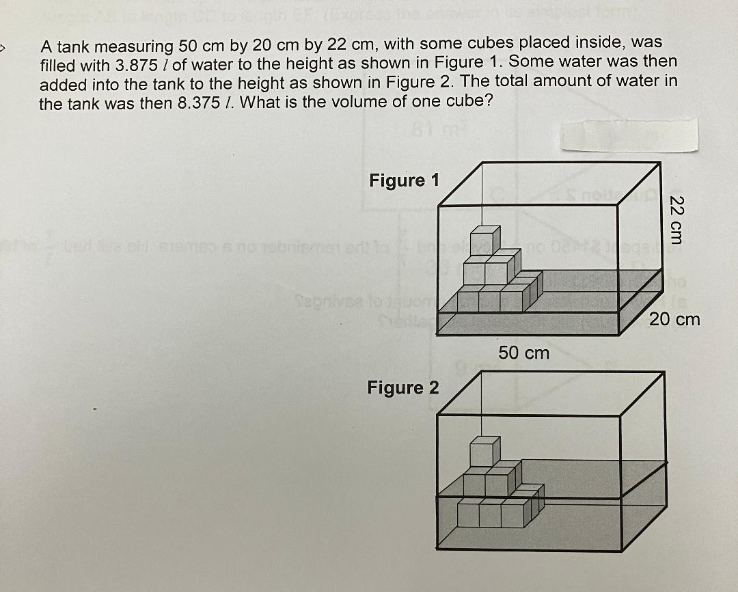# QuestionIs my working correct?

Actual amt of water to fill 2nd layer = 8.375 – 3.875 = 4.5 l

Difference between 1st and 2nd layer = 4.5 – 3.875 = 0.625 l (difference is 5 cubes less)

Vol of each cube = 0.625 / 5 cubes = 0.125 l